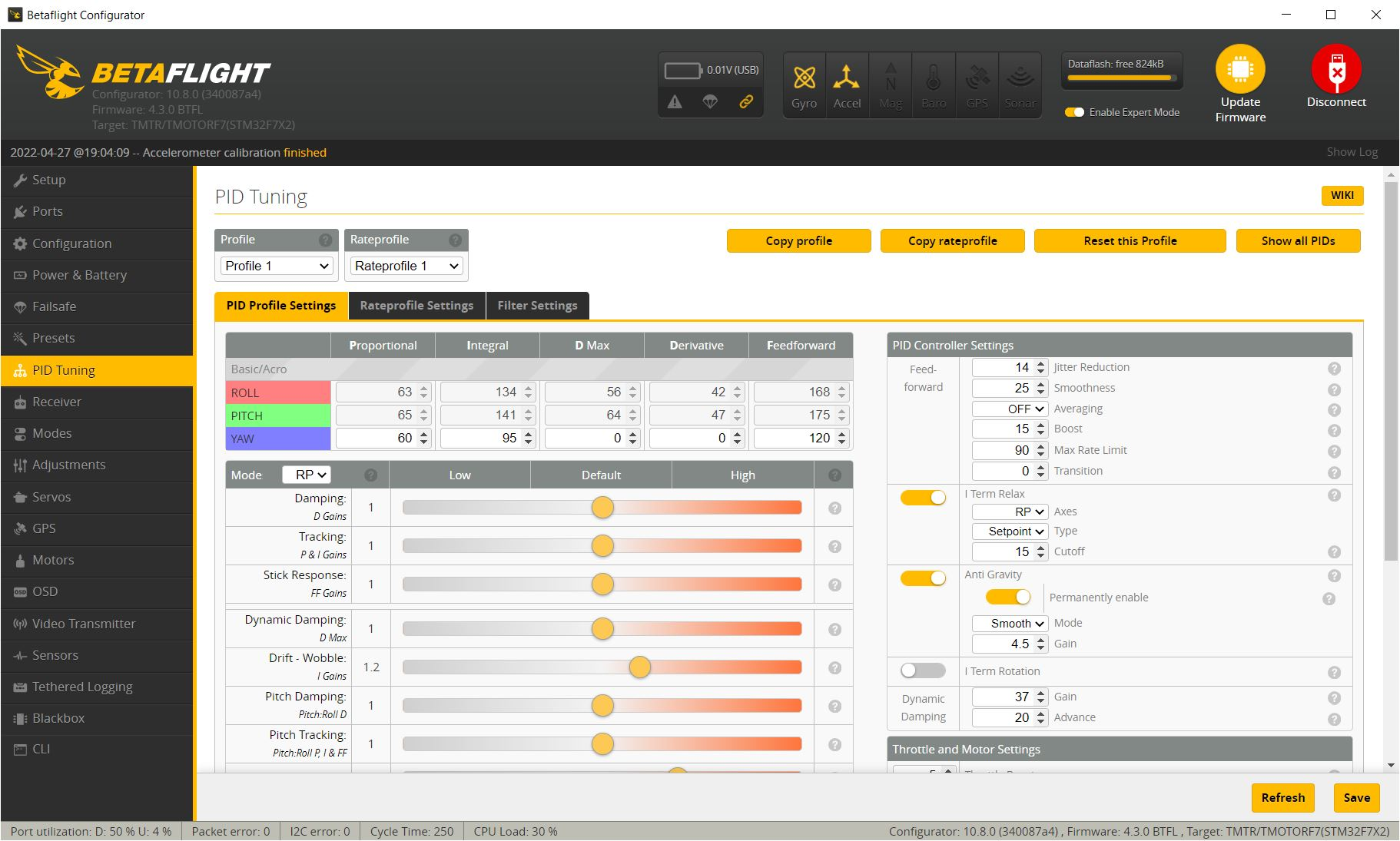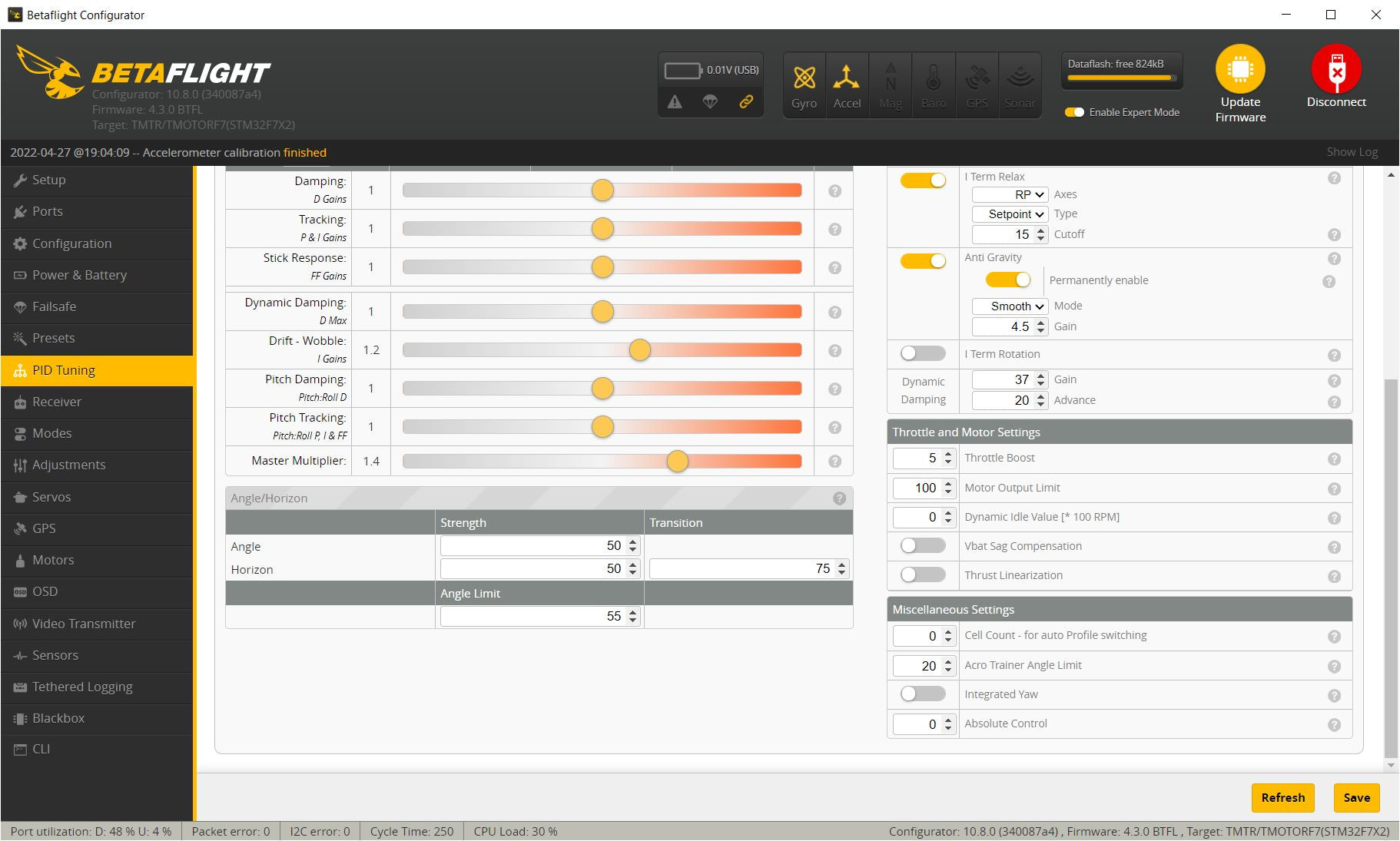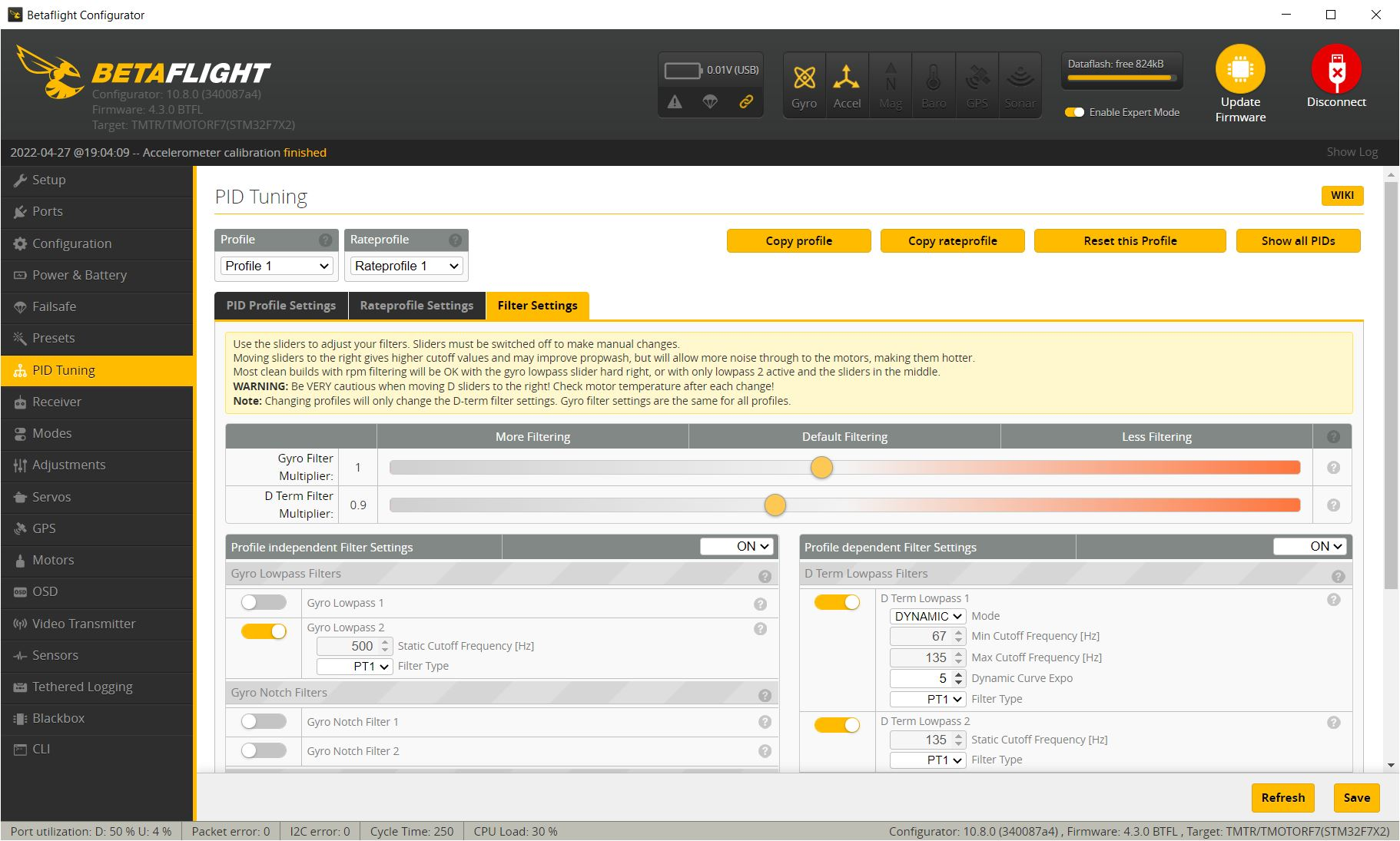# PIDS# Filters### CLI Input.# profile 0set dterm_lpf1_dyn_min_hz = 67set dterm_lpf1_dyn_max_hz = 135set dterm_lpf1_static_hz = 67set dterm_lpf2_static_hz = 135set anti_gravity_gain = 4500set p_pitch = 65set i_pitch = 141set d_pitch = 64set f_pitch = 175set p_roll = 63set i_roll = 134set d_roll = 56set f_roll = 168set p_yaw = 60set i_yaw = 95set d_min_roll = 42set d_min_pitch = 47set feedforward_jitter_factor = 14set simplified_pids_mode = RPset simplified_master_multiplier = 140set simplified_i_gain = 120set simplified_dterm_filter_multiplier = 90